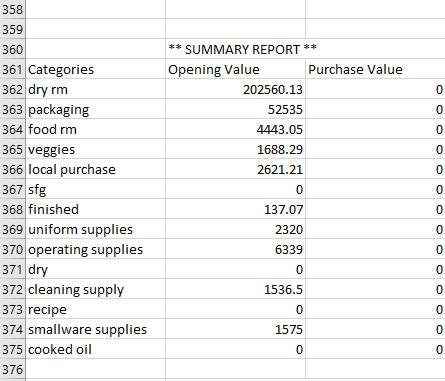# Delete the data from excel from ** SUMMARY REPORT ** to end of the SheetDelete the data from excel cell contains (cell rance changed dyanamically) ** SUMMARY REPORT ** to end of the Sheet and also find the attaached snap for your reference.

@Santhiya_0412

For this first you need to find the Index of the value where it is
Check below expression to get the Index where the value is

Index = dt.AsEnumerable().Select(Function(row, index) index).Where(Function(i) dt.Rows(i).Field(Of String)(“Name”) = “SUMMARY REPORT”).FirstOrDefault()

Now Once you get that Index then you can write below as

dt.AsEnumerable().Skip(Index).CopytoDatatable